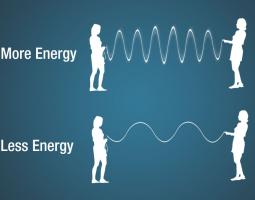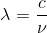# 3.2 The Electromagnetic Spectrum

Elizabeth Johnson and Juhong Christie Liu

# The Electromagnetic Spectrum

The video below gives an overview of the electromagnetic spectrum:

Figure 3.2.1. Overview of the Electromagnetic Spectrum. Science at NASA.

# Frequency and Wavelength

The number of crests that pass a given point within one second is described as the frequency of the wave. One wave—or cycle—per second is called a Hertz (Hz), after Heinrich Hertz who established the existence of radio waves. A wave with two cycles that pass a point in one second (as shown for the top wave in Figure 3.2.2) has a frequency of 2 Hz.

Electromagnetic waves have crests and troughs similar to (but not identical in behavior to) those of ocean waves. The distance between crests is the wavelength.

The figure below shows the wavelength (meters) and energy (Hz) ranges for different types of electromagnetic energy:

After watching the video and examining the figure above, answer these questions:

Guided Inquiry

# Visible Light

The visible light region is divided into color regions (Figure 3.2.4).  Color, and color changes, are important for many techniques including identification of minerals under thin section and Raman spectroscopy.

Guided Inquiry

# Energy

An electromagnetic wave can also be described in terms of its energy—in units of measure called electron volts (eV). An electron volt is the amount of kinetic energy needed to move an electron through one volt potential. Moving along the spectrum from long to short wavelengths, energy increases as the wavelength shortens. Consider a jump rope with its ends being pulled up and down. More energy is needed to make the rope have more waves.Figure 3.2.5. Higher and lower energy waves. From National Aeronautics and Space Administration, Science Mission Directorate. (2010). Anatomy of an Electromagnetic Wave. Retrieved March 9, 2019, from NASA Science website: http://science.nasa.gov/ems/02_anatomy

Guided Inquiry

3.2.5. Does a wave with a high frequency have a high energy?  Explain your answer.

Following from the answer above, we can write an equation to describe the relationship between wavelength and frequency as:

Equation 3.2.1              c = νλ

The speed of light (electromagnetic energy) is a constant c = 3.00 × 108 m/s.  Wavelength is represented by lambda (λ), and frequency is represented by the Greek letter “nu” or ν.  Sometimes “f” is used to replace ν.

Guided Inquiry

3.2.6. Calculate the wavelength of a 1530-kHz AM radio signal.

Solution
Rearranging gives:For the ν = 1530 kHz AM radio signal:
λ=3.00×108 m/s / 1530×103 cycles/s =196 m

# Summary

The electromagnetic spectrum is divided into different categories of light energy: gamma rays, X-rays, ultraviolet, visible, and infrared light, microwaves, and radio waves.  The energy and wavelength of electromagnetic energy changes across the spectrum, with gamma rays having short wavelength and highest energies, and radio waves having longest wavelengths and lowest energies.  Humans can detect only a narrow range of electromagnetic energy with our eyes, called visible light.  Energy and frequency of light are positively correlated with each other, but are negatively correlated with wavelength.

## References

Lumen Learning Physics Textbook: https://courses.lumenlearning.com/physics/

National Aeronautics and Space Administration, Science Mission Directorate. (2010). Introduction to the Electromagnetic Spectrum. Retrieved March 9, 2019, from NASA Science website: http://science.nasa.gov/ems/01_intro

National Aeronautics and Space Administration, Science Mission Directorate. (2010). Anatomy of an Electromagnetic Wave. Retrieved March 9, 2019, from NASA Science website: http://science.nasa.gov/ems/02_anatomy

OpenStax, College Physics. OpenStax CNX. Mar 4, 2019 http://cnx.org/contents/031da8d3-b525-429c-80cf-6c8ed997733a@14.48

ScienceAtNASA (May 6, 2010) Tour of the EMS 01- Introduction. https://youtu.be/lwfJPc-rSXw